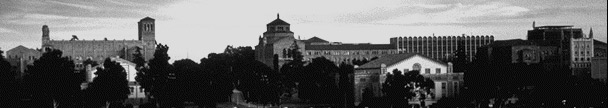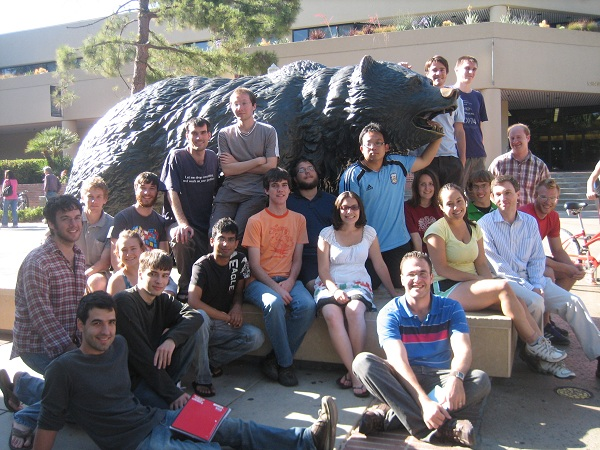UCLA Logic Center# 2010 Undergraduate Summer School

## (This is the 2010 page. Are you looking for the current Summer School?)

The UCLA Logic Center held a three-week summer school for undergraduates, from Monday July 5, to Friday July 23, 2010. (Summer school flyer, 8.5 x 11 inches, PDF format.)

The goal of the center's summer schools is to introduce future mathematicians to central results and techniques from mathematical logic. Courses are very intensive, designed to assume little if any prior experience with logic, yet reach highly advanced, graduate level material, within three weeks. They serve as good introduction to the kind of research work that students of mathematics can expect in graduate school.

Thanks to an NSF grant and Logic Center support we were able to provide admitted students with a stipend of \$3000, travel allowance up to \$500, and dormitory housing at UCLA for no charge.

Courses in the summer school meet daily for two hours of lecture, and one hour of guided problem solving. Students are asked to select two of the three courses offered. In addition to the six daily hours of course work, there are lectures on topics of current research, social events, and planned outings in the area.### Topics in 2010:

Computability and complexity
Instructor: Henry Towsner

This course will discuss some of the most important notions of solvability for mathematical problems. The first notion is given by the computable functions—simply those operations which can be carried out by an imaginary computer, given "only" unlimited time and space. With a rigorous notion of which problems can be solved, it is possible to show that many interesting problems can not be. It is also possible to make precise the idea that one unsolvable problem is more difficult than another, and we will study the hierarchy of solvability this gives. Many would object that when a solution requires a computer as big as the universe to calculate for as long as the history of time, perhaps the problem isn't really solvable. We will study the class P of problems which can be solved by a computable function with a polynomial bound on the computation time. We will see that many statements about computable functions correspond to natural statements about polynomially computable functions, but that the two notions have very different properties. We will see the easily-solved computable analog of the famous P/NP problem, and prove some theorems that show why P/NP is much harder. We will conclude with a third notion—random programs which quickly solve the problem with high probability, a notion that has become increasingly important in modern mathematics.

Determinacy and set theory
Instructor: Justin Palumbo

Principles of determinacy state the existence of winning strategies in certain two-player games of infinite length. They were initially considered in the '30s and '50s in connection with various topological properties; for example the famous Banach-Mazur game relates determinacy to the Baire property and other games relate determinacy to the perfect set property and Lebesgue measurability. Despite their somewhat humble beginnings, these innocuous-looking principles are today understood to have surprisingly deep connections with set theory at the highest and lowest levels of the infinite. At the high end they are intricately connected to large cardinal axioms, and at the low end they can be used to develop a deep structure theory for definable sets of reals. In this course we will explore the effects that determinacy hypotheses have on the structure of the set theoretic universe and especially on the real line, while simultaneously introducing students to basic techniques and tenets of descriptive set theory. We will also investigate the extent to which determinacy can be realized just on the basis of the classical mathematical axioms.

O-minimal structures
Instructor: Isaac Goldbring

In real algebraic geometry one is forced to consider subsets of Rn defined by polynomial equalities and inequalities; such sets are called semialgebraic. The semialgebraic sets possess a rather "tame" topology. For example, by the classical Tarski-Seidenberg Theorem, the projection of a semialgebraic set is also semialgebraic, and each semialgebraic set has only finitely many connected components, each of them semialgebraic. These and other finiteness theorems of a similar nature prompted Grothendieck to propose a search for wider classes of sets with the same tame topological properties. O-minimality provides such classes. Definable sets in o-minimal structures satisfy nearly all finiteness theorems true for semialgebraic sets, and o-minimal structures can yield richer classes of sets, for example including sets defined by exponential polynomial equalities and inequalities (Wilkie, 1996). In this course we will discuss the geometry of definable sets in o-minimal structures and model-theoretic properties of o-minimal theories. Among other things we will prove the finiteness theorems above, and study asymptotic growth behaviors of definable functions in o-minimal expansions of the real field.